# Initialize Clustering Model (DBSCAN) VI

LabVIEW 2018 Analytics and Machine Learning Toolkit Help

Edition Date: July 2018

Part Number: 377059B-01

»View Product InfoDownload Help (Windows Only)

Owning Palette: Clustering VIs

Requires: Analytics and Machine Learning Toolkit

Initializes the hyperparameters of the density-based spatial clustering of applications with noise (DBSCAN) algorithm. You can either directly set the hyperparameters or specify multiple values for each hyperparameter. If you specify multiple values for each hyperparameter, the Train Clustering Model VI uses grid search to find the optimal set of hyperparameters.

Examples

Use the pull-down menu to select an instance of this VI.

 Select an instance Set ParametersSearch Parameters

## Set Parameters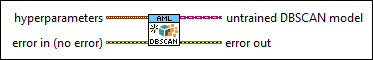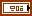hyperparameters specifies hyperparameters for the DBSCAN model.max distance specifies the maximum radius for a sample to form a neighborhood. The default is 1.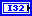min samples specifies the minimum number of samples that need to be in the neighborhood of a core sample. The default is 5.p specifies the power of the Minkowski metric that this VI uses to calculate distances between points. The default is 2. The following equation defines the Minkowski metric: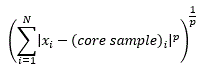where [x1, x2, …, xN] is the input feature vector and [core sample1, core sample2, …, core sampleN] is the core sample feature vector.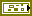error in describes error conditions that occur before this node runs. This input provides standard error in functionality.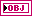untrained DBSCAN model returns the initialized DBSCAN model for training.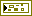error out contains error information. This output provides standard error out functionality.

## Search Parameters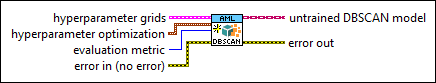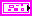hyperparameter grids specifies multiple values for each hyperparameter of the DBSCAN model.max distance specifies the maximum distance between two samples for them to share the same neighborhood.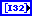min samples specifies the minimum number of samples in a neighborhood for a point to be a core point.p specifies the power of the Minkowski metric that this VI uses to calculate distances between points. The following equation defines the Minkowski metric:where [x1, x2, …, xN] is the input feature vector and [core sample1, core sample2, …, core sampleN] is the core sample feature vector.hyperparameter optimization specifies the method of optimization to determine the optimal hyperparameter settings.hyperparameter search method specifies the method to search for the optimal set of hyperparameters.

 0 Exhaustive search (default)—Tests all possible hyperparameter combinations in the training process. This method is reliable but time-consuming. 1 Random search—Tests a random subset of the hyperparameter combinations in the training process. This method is faster.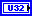number of searchings specifies the number of hyperparameter combinations that this VI selects to train the model. The default is 1. This input is valid only if hyperparameter search method is Random search.evaluation metric specifies the criterion to evaluate the trained clustering model.

 0 Davies Bouldin Index—Evaluates the clustering model using the Davies Bouldin Index metric. The lower the value of metric, the better the compactness and separation of the clustering model. 1 Dunn Index (default)—Evaluates the clustering model using the Dunn Index metric. The higher the value of metric, the better the compactness and separation of the clustering model. 2 Jaccard Index—Evaluates the clustering model using the Jaccard Index metric. The lower the value of metric, the better the separation of the clustering model. 3 Rand Index—Evaluates the clustering model using the Rand Index metric. The lower the value of metric, the better the separation of the clustering model.error in describes error conditions that occur before this node runs. This input provides standard error in functionality.untrained DBSCAN model returns the initialized DBSCAN model for training.error out contains error information. This output provides standard error out functionality.

## Examples

Refer to the following VIs for examples of using the Initialize Clustering Model (DBSCAN) VI:

• Clustering (Set Parameters, Training) VI: labview\examples\AML\Clustering
• Clustering (Search Parameters, Training) VI: labview\examples\AML\Clustering